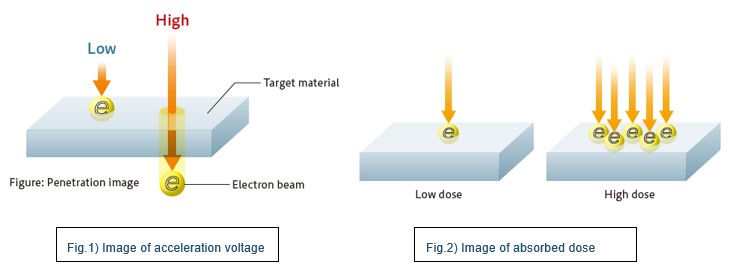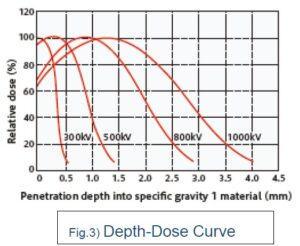## Useful information for Electron Beam

### Effect of electron beams on Acceleration Voltage and thickness direction

Posted date:March 20, 2023Last updated:March 20, 2023

This page describes the concept of how electron beams affect the thickness direction of materials and how to select Acceleration Voltage.

#### Two key parameters in electron irradiation

There are two key parameters in electron irradiation: acceleration voltage and absorbed dose.
①Acceleration voltage
The higher the acceleration voltage, the faster the speed of the electrons and the more they can penetrate (process) even thicker samples.(Fig.1)
Absorbed dose
It corresponds to the amount of energy (number of electrons) given to the object and is a parameter that is adjusted according to the degree of processing required.(Fig.2)
In this article, the acceleration voltage is presented in detail in terms of the effect of the electron beam on the thickness direction.#### Electron influence in the direction of thickness = Penetration Power！

The influence of electrons on the thickness direction of a material is called the penetration power. The penetration power at a given acceleration voltage is expressed as a penetration power curve (Depth-Dose Curve), as shown in Fig. 3.The vertical axis is the relative dose, which expresses the amount of energy absorbed by the substance in relative values. The horizontal axis is the depth of penetration into the substance with specific gravity 1.
For example, if a material with specific gravity 1 is irradiated at an acceleration voltage of 300 kV, the dose at the surface of the sample (horizontal axis 0 mm) is almost 100%, but the dose decreases as it enters the interior of the material and is less than 10% at a position 0.5 mm from the surface.
For a material with specific gravity ρ, the penetration power is 1/ρ. The higher the specific gravity, the harder it is for electrons to penetrate, and conversely, the lower the specific gravity, the easier it is for electrons to penetrate, so for a specific gravity of 2, the value on the horizontal axis is one-half, and for a specific gravity of 0.5, it is twice the penetration capacity for that material. As the acceleration voltage increases, the graph shows a peak in the relative dose at a slightly deeper level than the surface. This is caused by the speed of theelectrons incident on the material being too fast, so that they pass through the material before giving it enough energy.

#### Method of selecting acceleration voltage

We recommend a thickness of 60% relative dose (80% for curing) as the processable thickness at that acceleration voltage.
For example, if you want to crosslink a 1 mm thick material with a specific gravity of 1, you should select an acceleration voltage of 800 kV or higher, as considered in Fig 3.
The key point here is that even within a 1 mm thickness, there will be a dose difference of approximately 40% between the surface (0 mm abscissa) and the back surface (1 mm abscissa).
If the results differ between the front and back surfaces, this dose difference may be the cause.
One method of reducing dose differences in the material is double-sided irradiation. Double-sided irradiation will be presented in a separate article at a later date.

#### Summary

The effect of the electron beam on the thickness direction is determined by the acceleration voltage; the higher the acceleration voltage, the thicker the sample can be processed. However, it is important to pay sufficient attention to the distribution of the dose in the thickness direction by referring to the depth-dose curve.
As the maximum acceleration voltage varies depending on the electron beam processing system, please inform us of the thickness and specific gravity of the material when considering irradiation tests. We will suggest the most suitable processing system and acceleration voltage.

［Inquiry］

NHV Corporation
Please inquire through the inquiry form.

«Previous Next»